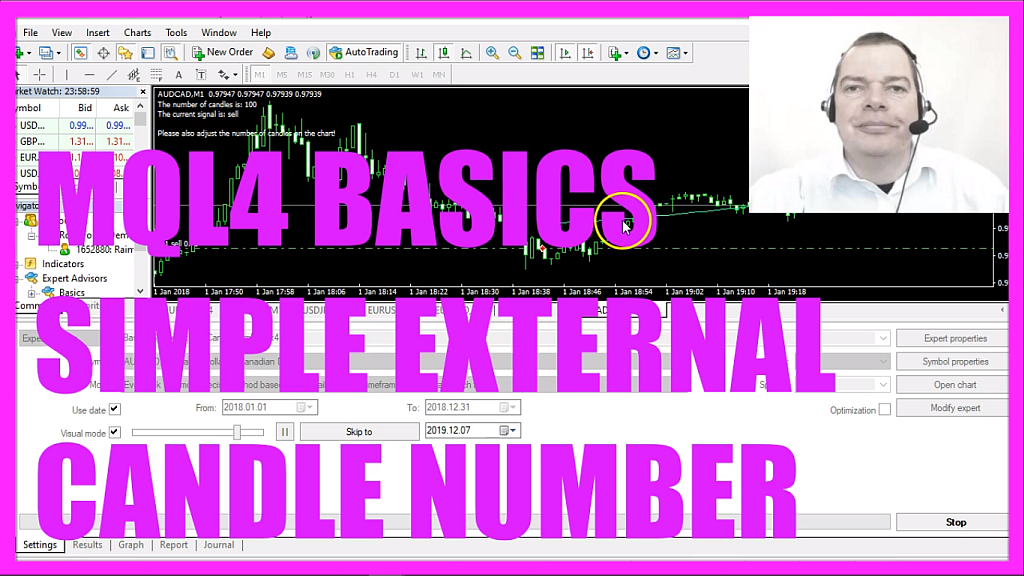In this video, we are going to create an expert advisor that is able to calculate a simple moving average based on an external variable for the number of candles. In this case, we are using one hundred candles to calculate the moving average, so let’s find out how to do that with mql4.
To get started please click on the little icon here or press F4 on your keyboard, now you should see the Metaeditor window and here you want to click on file, new file, expert advisor from template, continue, I will call this file simple external candle number, click on continue, continue and finish.
Now you can delete everything above the on tick function and the two comment lines here, we start by creating an external integer value called number of candles.
The first value will be one, we will change that later and the modifier extern is used to create something that can be adjusted without the need to change the source code.
Inside of the on tick function we start by creating a string variable for the signal, we don’t assign any value here because this is going to be calculated later.
To calculate the moving average we use the integrated ima function for the current symbol on the chart and the currently selected period on that chart.
We want to calculate it for the number of candles that we will define, this parameter and the last one have the value zero because we don’t want to use any shift values. This one is mode underscore sma – all in capital letters – that stands for simple moving average, we are going to calculate the result based on the close price and if the moving average value is below the current close price that would be a buy signal so we assign the word buy to our signal.
Otherwise, if the moving average value is bigger than the current close price that would be a sell signal and now we assign the word sell to our signal.
And when the signal is buy and orders total has a return value of zero, or in other words, we have no open orders that’s when we use order send to buy ten micro lot.
Otherwise, if the signal equals sell and we have no open orders that’s when we use order send to sell ten micro lot.
Finally, we use the comment function to create an output on the chart, it will output the number of candles is, followed by the calculated value for the number of candles.
In a new row, we want to see the current signal is followed by the calculated signal and below we also want to output a hint: Please also adjust the number of candles on the chart!